# 2.2 Theoretical Background

The structure of CNTs has been explored early by high resolution transmission electron microscopy techniques yielding direct confirmation that the CNTs are seamless cylinders derived from the honeycomb lattice representing a single atomic layer of crystalline graphite, called a graphene sheet. The structure of a SW-CNT is conveniently explained in terms of its one-dimensional unit cell, defined by the vectors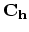and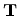as shown in Fig. 2.2.

The circumference of any CNT is expressed in terms of the chiral vector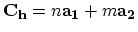which connects two crystallographically equivalent sites on a two-dimensional graphene sheet . The construction in Fig. 2.2 depends uniquely on the pair of integers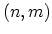which specify the chiral vector. The chiral angle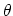is defined as the angle between the chiral vectorand the zigzag direction (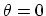). Three distinct types of CNT structures can be generated by rolling up the graphene sheet into a cylinder as shown in Fig. 2.3. The zigzag and armchair CNTs correspond to chiral angles ofand°, respectively, and chiral CNTs correspond to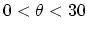°. The intersection of the vector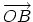(which is normal to) with the first lattice point determines the fundamental one-dimensional translation vector. The unit cell of the one-dimensional lattice is the rectangle defined by the vectorsand.

The cylinder connecting the two hemispherical caps of the CNT (see Fig. 2.3) is formed by superimposing the two ends of the vectorand the cylinder joint is made along the two linesand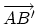in Fig. 2.2. The linesandare both perpendicular to the vectorat each end of. In thenotation for, the vectors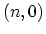or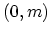denote zigzag CNTs, whereas the vectorscorrespond to chiral CNTs . The CNT diameter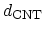is given by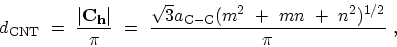(2.1)

where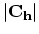is the length ofand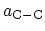is the C-C bond length (1.42 Å). The chiral angleis given by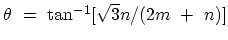. For the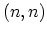armchair CNT° and for thezigzag CNT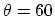°. From Fig. 2.2 it follows that if one limitsto the range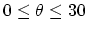°, then by symmetry,for a zigzag CNT. Both armchair and zigzag CNTs have a mirror plane and thus are considered achiral. Differences in the CNT diameterand chiral anglegive rise to different properties of the various CNTs. The number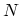of hexagons per unit cell of a CNT, specified by integers, is given by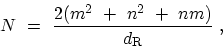(2.2)

where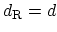if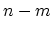is not a multiple of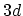, and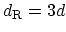ifis a multiple of, and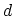is defined as the greatest common divisor (gcd) of. Each hexagon in the honeycomb lattice contains two carbon atoms. The unit cell area of the CNT istimes larger than that for a graphene layer and consequently the unit cell area for the CNT in reciprocal space is correspondingly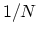times smaller. Table 2.2 provides a summary of relations useful for describing the structure of SW-CNTs [12,22].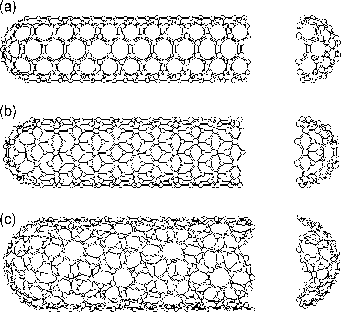Table 2.2: Structural properties for CNTs .
Symbol Description Formula
a length of unit vectors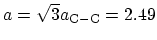Å,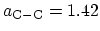Å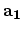,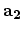unit vectors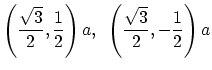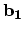,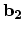reciprocal lattice vectors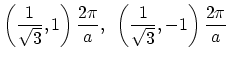chiral vector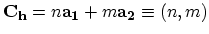,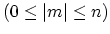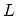length of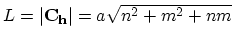diameter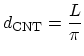chiral angle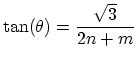gcd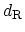gcd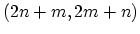translational vector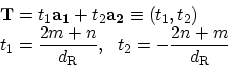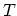length ofnumber of hexagons in the unit-cell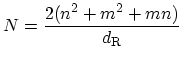M. Pourfath: Numerical Study of Quantum Transport in Carbon Nanotube-Based Transistors